#### Excel八大经典函数组合整理篇

1、IF + And

【例】如下图所示，在C列设置公式，如果A列值小于500且B列值为未到期，则返回”补款“，否则显示为空。

=IF(AND(A2<500,B2="未到期"),"补款","")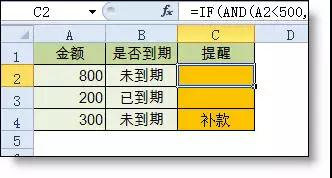2、Index + Match

【例2】如下图所示，要求根据月份和费用项目，查找金额

E10公式：

=INDEX(B2:G6,MATCH(B10,\$A\$2:\$A\$6,0),MATCH(A10,\$B\$1:\$G\$1,0))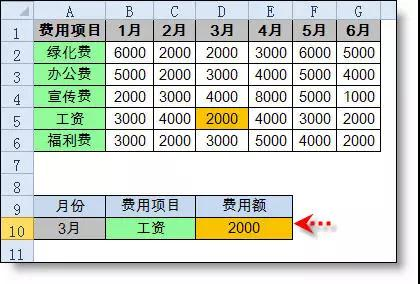3、Iferror + Vlookup

【例】如下图所示，要求根据产品名称在上表中查找单价，如果产品不存在则显示为空白

B9公式：

=IFERROR(VLOOKUP(A8,\$A\$1:\$D\$5,3,0),"")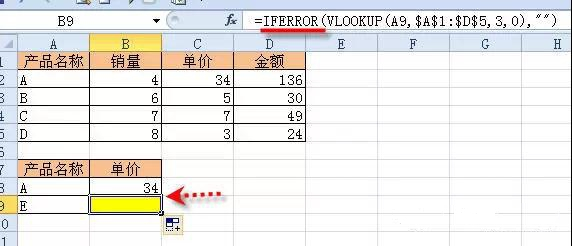4、Mid + Find

【例】在个人信里截取出年龄

B2公式

=MID(A2,FIND(" ",A2)+1,9)5、Left + lenB + Len

=LEFT(A2,LENB(A2)-LEN(A2))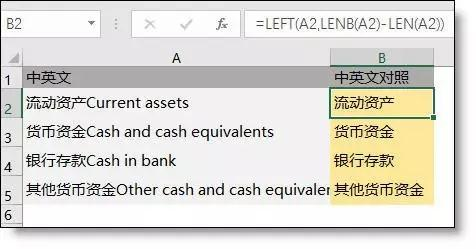6、index + Small + Row

【例】如下图所示，要求在F列查找“张明城”的个人消费记录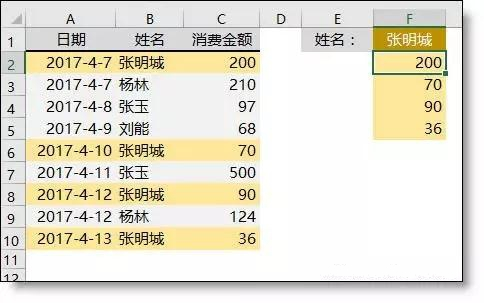F2公式

{=INDEX(C:C,SMALL(IF(B\$2:B\$10=F\$1,ROW(\$2:\$10)),ROW(A1)))}

IF(B\$2:B\$10=F\$1,ROW(\$2:\$10))：如果B列的姓名和F1的姓名相同，就返回它的行号。不相同的返回FALSE

Row(a1)：是返回A1的行号1，如果向下复制会变为 Row(a2)，返回2，其实用它的目的是当公式向下复制时可以生成序号：1，2，3...然后取符合条件的第1个行号，第2个行号...

SMALL(): 从符合条件的行号中从小到大，逐个提取符合条件的行

INDEX() :根据取得的行号从C列提取值

{ }：数组公式(含有逐一运算的公式)需要按ctrl+shift+enter 输入大括号(一定要是自动生成的，不能手输入大括号)。

7、Sum + Offset + Count

【例】在D2单元格返回B列最近7天的销量

D2公式：

=SUM(OFFSET(B1,COUNTA(B:B)-7,0,7,1))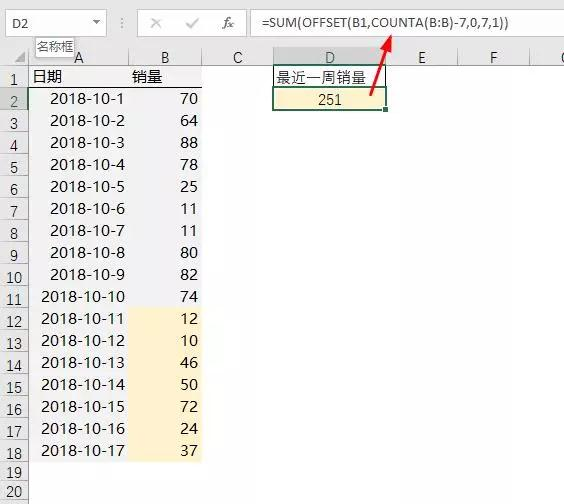8、Sumproduct + Countif

【例】：统计B列的客户数量

=SUMPRODUCT(1/COUNTIF(B2:B19,B2:B19))